Java Games: Flashcards, matching, concentration, and word search.# graphs and linear equations

Matching simple linear graphs to their equations

AB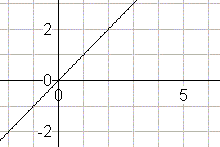y = x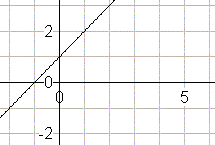y = x + 1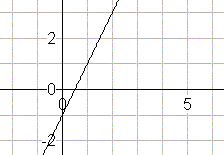y = 2x - 1y = 2x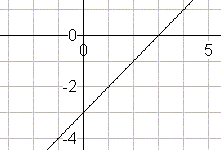y = x - 3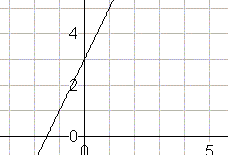y = 2x +3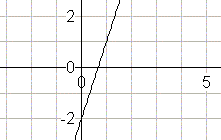y = 3x - 2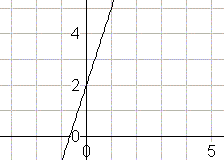y = 3x + 2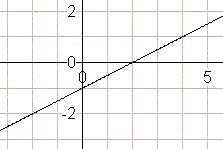0.5x -1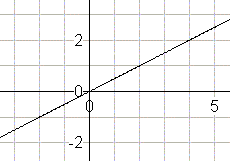y = 0.5x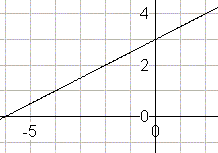y = 0.5x + 3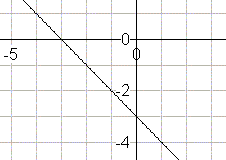y = -x - 3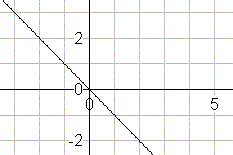y = -x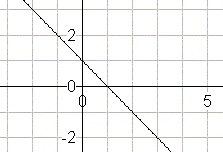y = -x + 1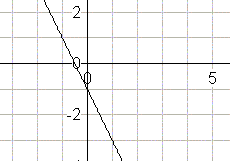y = -2x - 1y = -2xThis activity was created by a Quia Web subscriber. Learn more about QuiaCreate your own activities